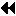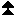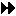All-Reduce

## All-ReduceUp: Global Reduction Operations Next: Reduce-Scatter Previous: Example of User-defined Reduce

MPI includes variants of each of the reduce operations where the result is returned to all processes in the group. MPI requires that all processes participating in these operations receive identical results.

MPI_ALLREDUCE( sendbuf, recvbuf, count, datatype, op, comm)
[ IN sendbuf] starting address of send buffer (choice)
[ IN count] number of elements in send buffer (integer)
[ IN datatype] data type of elements of send buffer (handle)
[ IN op] operation (handle)
[ IN comm] communicator (handle)

int MPI_Allreduce(void* sendbuf, void* recvbuf, int count, MPI_Datatype datatype, MPI_Op op, MPI_Comm comm)

MPI_ALLREDUCE(SENDBUF, RECVBUF, COUNT, DATATYPE, OP, COMM, IERROR)
<type> SENDBUF(*), RECVBUF(*)
INTEGER COUNT, DATATYPE, OP, COMM, IERROR

Same as MPI_REDUCE except that the result appears in the receive buffer of all the group members.

The all-reduce operations can be implemented as a reduce, followed by a broadcast. However, a direct implementation can lead to better performance. ( End of advice to implementors.)

```

A routine that computes
the product of a vector and an array that are distributed across a
Predefined reduce operations
).

SUBROUTINE PAR_BLAS2(m, n, a, b, c, comm)
REAL a(m), b(m,n)    ! local slice of array
REAL c(n)            ! result
REAL sum(n)
INTEGER n, comm, i, j, ierr

! local sum
DO j= 1, n
sum(j) = 0.0
DO i = 1, m
sum(j) = sum(j) + a(i)*b(i,j)
END DO
END DO

! global sum
CALL MPI_ALLREDUCE(sum, c, n, MPI_REAL, MPI_SUM, 0, comm, ierr)

! return result at all nodes
RETURN

```Up: Global Reduction Operations Next: Reduce-Scatter Previous: Example of User-defined Reduce